FUTURE VALUE OF INVESTMENTS

INTRODUCTION

This describes a class of problems in which we want to calculate the interest rate “x” which will yield a desired amount of money “D” at some time in the future.   The means to this end involve both an initial lump sum payment “A” as well as subsequent periodic payments “M”.

Note that if instead of a yearly interval we wanted something shorter, then we would simply divide the yearly interest rate by the number of intervals in a year and would multiply the number of years by that same number.  For instance if we wanted to change from yearly to monthly intervals we would make the following transformations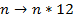FUTURE VALUE OF SINGLE LUMP SUM PAYMENT

If we make an initial lump sum payment of “A” then the desired value “D” after “n” years at an annual interest rate of “x” is given as follows (see Appendix on Compound Interest):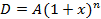In this case the required interest rate “x” for “n” years has a simple closed form solution as expressed in the following equation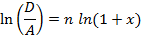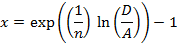FUTURE VALUE OF PERIODIC PAYMENTS

If we make a payment “M” and then repeat this payment every year for “n” years at an annual interest rate of “x”, where the last payment is made at the very end and thus collects no interest, then the desired value “D” is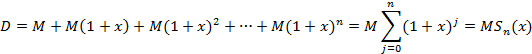Note that we can approximate the desired value as (see Appendix on Expansion of the Sum, Sn)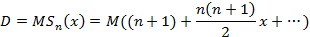Using this simplification we can get an initial guess for the interest rate as follows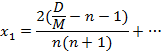Unfortunately a closed form solution for the interest rate “x” is not possible, so we use successive approximations using the Newton-Raphson method as follows.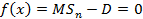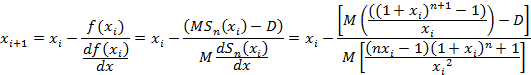which is easily reduced to the simple expression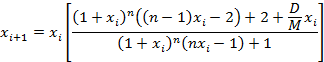FUTURE VALUE OF AN INITIAL LUMP SUM AND PERIODIC PAYMENTS

If we initially make a lump sum payment “A” and then on the next year and subsequent anniversaries make a payment “M” for “n” years at an annual interest rate of “x” (noting the payment in the last year does not collect any interest), then the desired value “D” is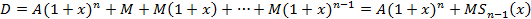The desired value can be approximated asand we can use this to get an initial guess for the interest rate as follows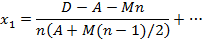And we must again use simplistic successive approximations to get the interest rate “x” as follows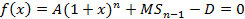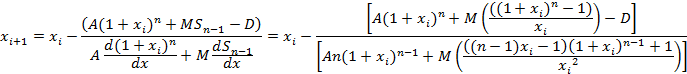which is slightly more complicated than before but still easily simplified to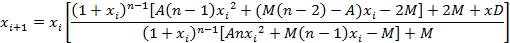APPENDIX: COMPOUND INTEREST

If we start with a lump sum of “A” and enjoy a yearly interest rate of “x”, then after one year we have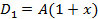And after two years we have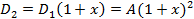And after three years we haveAnd generalizing to “n” years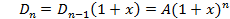APPENDIX: EXPANSION OF THE SUM Sn

The expression for a sum of a base to increasing powers is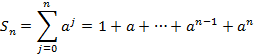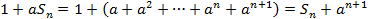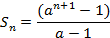Let us recall the binominal theorem as follows (whose coefficients come from Pascal’s Triangle or from the combinatorial expression)where the combinatorial for the number of ways one can choose “s” distinguishable objects from a larger collection of “r” objects is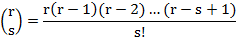Note that in the combinatorial, there are “s” values on both the top and bottom and the expression when “s” is zero is defined to be one (i.e. there is only one way to choose nothing).

Then we can expand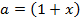as a power series and also we can expand the sum Sn as a power series: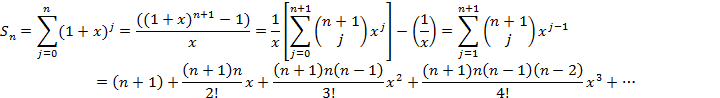APPENDIX: DERIVATIVE OF THE SUM Sn

The derivative of a function to a power is as follows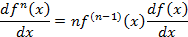If we define the function f(x) = (1+x), thenAnd if we recall that the derivative of the division of two functions is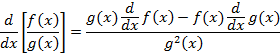then for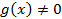, the derivative of the sum of a base to increasing powers, Sn,  is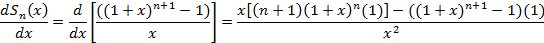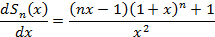APPENDIX: AN APPROXIMATE METHOD FOR FINDING ROOTS

In the Newton-Raphson method we use a series of successive approximations, which are quadratically not linearly convergent, to approximate the root of a function.  Please recall that the root is the value of the independent variable “xn” for which the function is zero.We start with an informed guess for the root “xi“ and, at that point, calculate the derivative as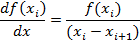The derivative is the tangent to the function at “xi“.  We extend the tangent to intercept the x-axis as shown below, so from our last guess of “xi” we get a better approximation “xi+1” of the actual root “xn”.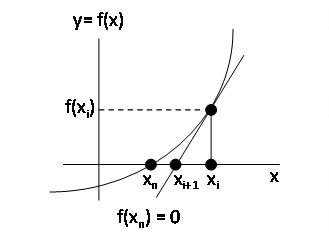The next best guess for the root is thus given by the following equation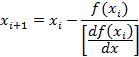We repeat this process multiple times so starting from an initial guess, x1, we get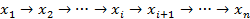After a few such iterations, we are for all practical purposes close enough as determined by how close the calculated value of the last approximation f(xi+1) is to zero.